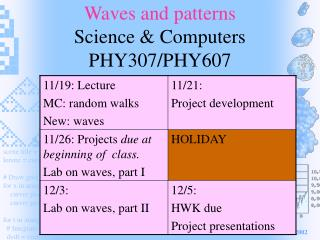DownloadDownload PresentationWaves and patterns Science & Computers PHY307/PHY607

# Waves and patterns Science & Computers PHY307/PHY607

Télécharger la présentation## Waves and patterns Science & Computers PHY307/PHY607

- - - - - - - - - - - - - - - - - - - - - - - - - - - E N D - - - - - - - - - - - - - - - - - - - - - - - - - - -
##### Presentation Transcript

1. Waves and patternsScience & ComputersPHY307/PHY607 PHY307, Fall 2002

2. HWK MC #2 • Random walks: the summation of random changes. • Bacteria moves “forward”, but buffeted randomly by surrounding molecules. • The DIRECTION accumulates random changes (a random walk on the sphere of directions.) • Stock prices: Nobel prize winning model (Black-Scholes) for estimating future values of stocks and options. • The PRICE accumulates random changes (multiplied by a random number.) PHY307, Fall 2002

3. from visual.graph import * from random import * stockchart = gcurve() price = 100.0 for week in range(50): growth = 0.9 + 0.25 * random() price *= growth stockchart.plot(pos=(week,price)) print `Final price is`, price

4. Lab MC #2 • Average displacement grows as the square root of the time (since average of the square of the position grew proportional to time.) Characteristic feature of a simple random walk. • The density of a diffusion created aggregate decreases as the aggregate grows: fractal structure with a dimension less than 3. PHY307, Fall 2002

5. Few coordinate systems • Projectiles. • Coordinates might be x, y, and z positions and x, y, and z velocities. • Planets. • Similar coordinates. • Get periodic orbits and chaos. PHY307, Fall 2002

6. Extended (many-coordinate) systems • Can’t be described by a few coordinates • (Often can for some purposes. For example, Earth is extended, but treated as a point in the solar system.) • “Many degrees of freedom” • Can exhibit quite complex phenomena, including periodic waves, pattern formation, chaotic waves, and turbulence. PHY307, Fall 2002

7. Waves I

8. Waves II

9. Waves II, movie

10. Weather

11. Pattern formation (Turing)

12. Why waves? • Exchange between neighboring positions of • energy • material • whatever else • Also, convert from one form to another • Example: Height of water to motion of water. PHY307, Fall 2002

13. Oscillation • Exchange of energy in a few coordinate system as an example (not a wave yet.) • In a spring, the energy of distortion, which depends on position, is exchanged with the energy of motion, which depends on velocity. • Python demo. • Change in position is velocity • Change in velocity (acceleration) is force/mass, with force = - k * (position – rest_position) PHY307, Fall 2002

14. Concepts and Procedures for Simulating Waves • Approximate continuous space by a grid. • Need a list of the values of the quantities (positions, velocities, chemical concentrations.) This list gives the values of the quantities at the grid points. • Need rules for how quantities affect their values at neighboring grid points. PHY307, Fall 2002

15. Example • Demo of slinkie. • How do we describe the motion and simulate it? • FOR CODE DEVELOPED DURING LECTURE, SEE Codes page • http://physics.syr.edu/courses/PHY307/Codes/WaveInClass.py PHY307, Fall 2002